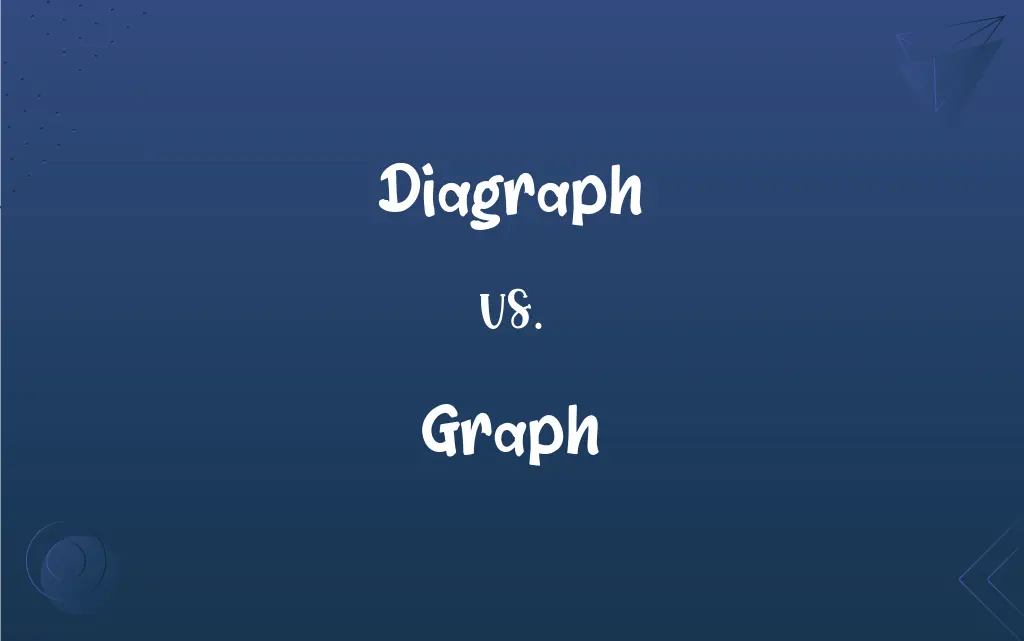# Diagraph vs. Graph## Diagraph and Graph Definitions

#### Diagraph

(dated) A drawing instrument that combines a protractor and scale.

#### Graph

A diagram that exhibits a relationship, often functional, between two sets of numbers as a set of points having coordinates determined by the relationship. Also called plot.

#### Diagraph

A drawing instrument, combining a protractor and scale.

#### Graph

A pictorial device, such as a pie chart or bar graph, used to illustrate quantitative relationships. Also called chart.

#### Graph

The spelling of a word.

#### Graph

Any of the possible forms of a grapheme.

#### Graph

A written character that represents a vowel, consonant, syllable, word, or other expression and that cannot be further analyzed.

#### Graph

To represent by a graph.

#### Graph

To plot (a function) on a graph.

#### Graph

A data chart (graphical representation of data) intended to illustrate the relationship between a set (or sets) of numbers (quantities, measurements or indicative numbers) and a reference set, whose elements are indexed to those of the former set(s) and may or may not be numbers.

#### Graph

(mathematics) A set of points constituting a graphical representation of a real function; (formally) a set of tuples $\left(x_1, x_2, \ldots, x_m, y\right)\in\R^\left\{m+1\right\}$, where $y=f\left(x_1, x_2, \ldots, x_m\right)$ for a given function $f: \R^m\rightarrow\R$. See also Graph of a function Category:en:Curves Category:en:Functions

#### Graph

(graph theory) A set of vertices (or nodes) connected together by edges; (formally) an ordered pair of sets $\left(V,E\right)$, where the elements of $V$ are called vertices or nodes and $E$ is a set of pairs (called edges) of elements of $V$. See also Graph (discrete mathematics)

#### Graph

(topology) A topological space which represents some graph (ordered pair of sets) and which is constructed by representing the vertices as points and the edges as copies of the real interval [0,1] (where, for any given edge, 0 and 1 are identified with the points representing the two vertices) and equipping the result with a particular topology called the graph topology.

#### Graph

A morphism $\Gamma_f$ from the domain of $f$ to the product of the domain and codomain of $f$, such that the first projection applied to $\Gamma_f$ equals the identity of the domain, and the second projection applied to $\Gamma_f$ is equal to $f$.

#### Graph

A graphical unit on the token-level, the abstracted fundamental shape of a character or letter as distinct from its ductus (realization in a particular typeface or handwriting on the instance-level) and as distinct by a grapheme on the type-level by not fundamentally distinguishing meaning.

#### Graph

(transitive) To draw a graph.

#### Graph

To draw a graph of a function.

#### Graph

A curve or surface, the locus of a point whose coördinates are the variables in the equation of the locus; as, a graph of the exponential function.

#### Graph

A diagram symbolizing a system of interrelations of variable quantities using points represented by spots, or by lines to represent the relations of continuous variables. More than one set of interrelations may be presented on one graph, in which case the spots or lines are typically distinguishable from each other, as by color, shape, thickness, continuity, etc. A diagram in which relationships between variables are represented by other visual means is sometimes called a graph, as in a bar graph, but may also be called a chart.

#### Graph

A drawing illustrating the relations between certain quantities plotted with reference to a set of axes

#### Graph

Represent by means of a graph;
Chart the data

#### Graph

Plot upon a graph